# Two Degree-of-Freedom PID Control for Setpoint Tracking

### Model Description

This example shows how to regulate the speed of an electric motor using two degrees-of-freedom PID control with set-point weighting. The model uses the PID Controller (2DOF) block. The model changes the setpoint values between 550 and 250 rpm. To convert the units to rad/s for use in the PID controller, the model uses a Signal Conversion block.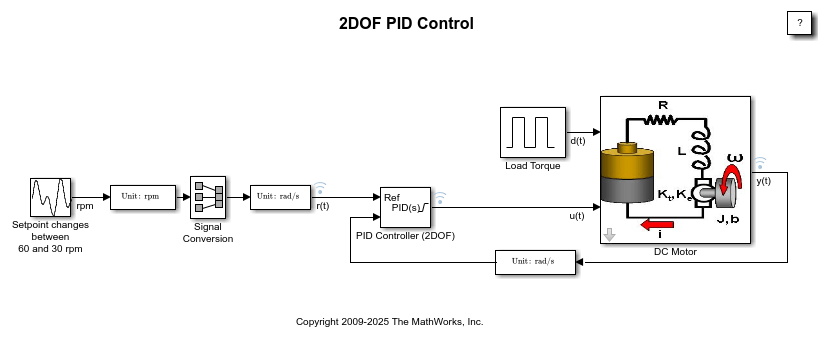Figure 1: Simulink model with two degree-of-freedom PID control of a DC motor.

The electric motor is an armature-controlled DC motor. Voltage input controls the shaft speed of the motor. Figure 2 shows the block diagram of the motor. The motor experiences a load torque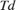(0-5 Nm).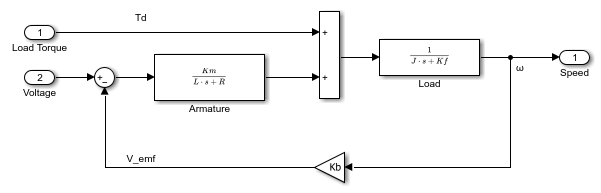Figure 2: Block diagram of the motor.

### Two Degree-of-Freedom PID Control

In contrast to the PID Controller block, the PID Controller (2DOF) block provides an extra degree of freedom, allowing you to weight the setpoint as it passes through the proportional action channel and the derivative action channel. Figure 3 shows the PID Controller (2DOF) block diagram.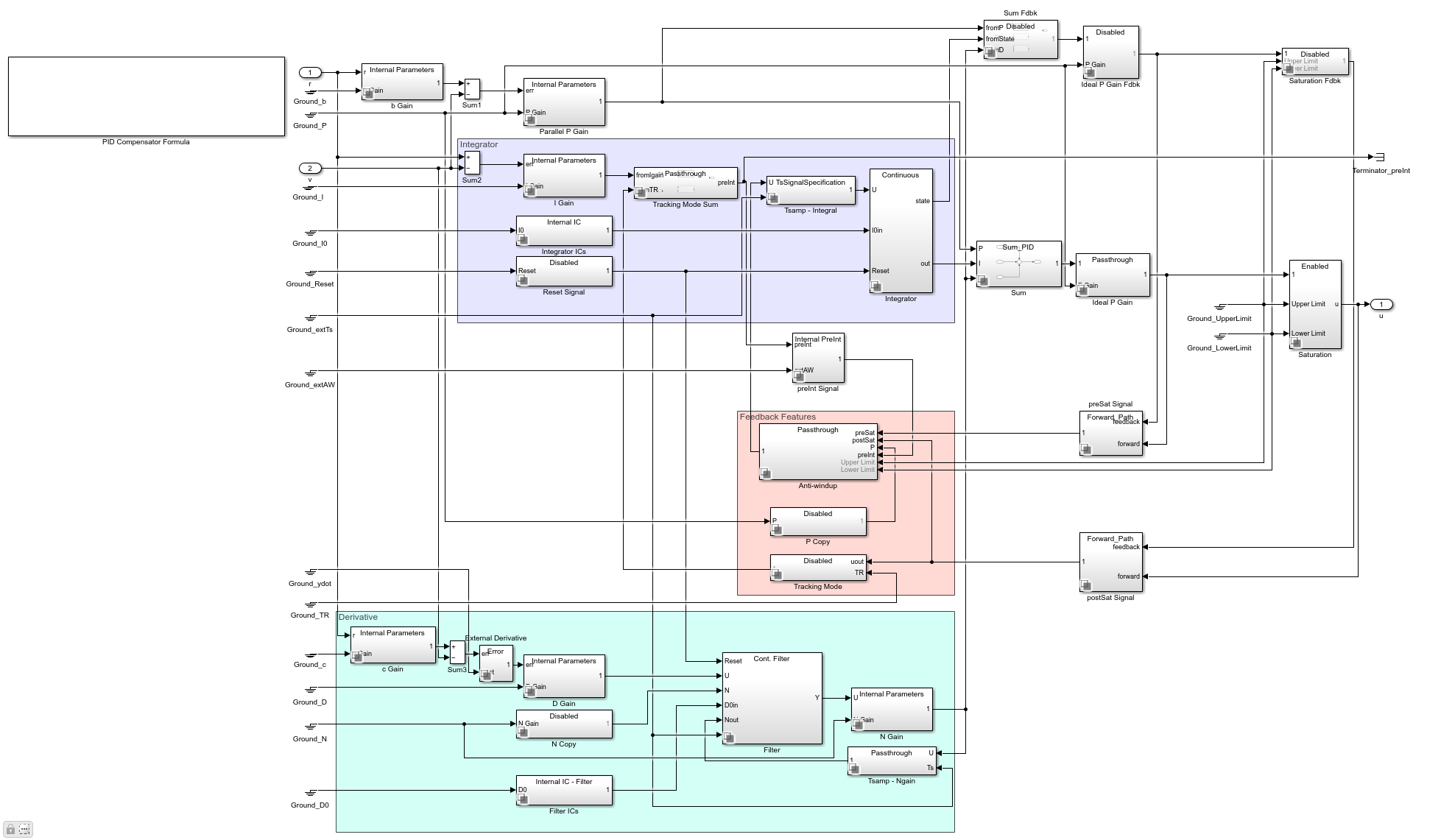Figure 3: Under-mask view of the PID Controller (2DOF).

As shown in Figure 3, the proportional action error signal is given by

``

The signal seen by the derivative action signal is given by

``

and the integral action signal is given by

``

In general, the setpoint weight c is set to 0. This prevents undesirable transients if the setpoint changes, an effect known as derivative kick. The setpoint b affects the overshoot performance of the controller. Generally, a small b value reduces overshoot. However, smaller b values can also result in slower response to setpoint changes. For more details on picking the right setpoint values, see Reference .

When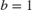and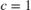, the behavior of the two degree-of-freedom PID controller is identical to a classical PID controller.

### Simulating with b = 1 and c = 1

Whenand, the behavior of the two degree-of-freedom PID controller is identical to a classical PID controller. The control signal, setpoint signal, and closed-loop response of the model are shown in Figure 4.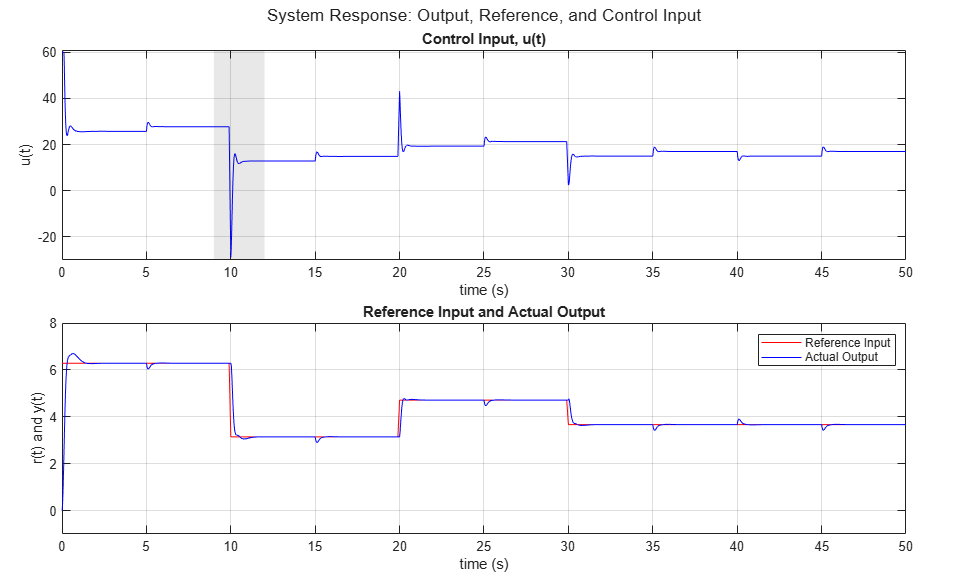Figure 4: Control signal, setpoint vs. measured output.

Figure 4 clearly shows the spikes in the control signal, caused by aggressive proportional and derivative response to the setpoint change. Modifying the b and c weights can make this response less aggressive, as shown next.

### Simulating with the b = 0 and c = 0

In this case, the two degree-of-freedom PID controller is known as I-PD where only the I action acts on the classical error signal, and the PD action acts only on the measured output.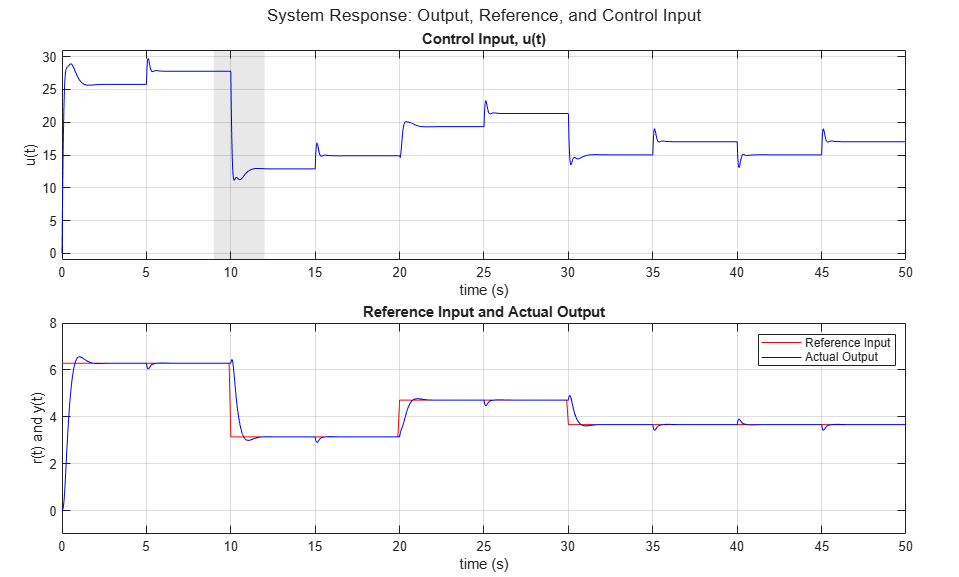Figure 5: Control signal, setpoint vs. measured output.

The simulation results show the absence of large transients in the control signal due to the sudden changes in the setpoint.

See Reference  for more information on how to chose b and c.

### Summary

The PID Controller (2DOF) block supports two degree-of-freedom PID control. Use this block for tracking complex setpoint profiles and moderating the impact of sudden setpoint changes on control signal transients. You can use the PID tuner in Simulink® Control Design™ to automatically tune all the gains (P, I, D, N, b, c) of the PID Controller (2DOF) block.

### References

1. K. Åström, T. Hägglund, Advanced PID Control, ISA, Research Triangle Park, NC, August 2005.